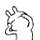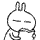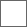### 看看i大吧主的真实面目暴露无遗

【你拿什么反相－－我们的反相者？】

dkcxp的那个贴子－－《什么样的人才是真正的【反相者】？》：“『说到底，【反相者】也就是正常追求科学真理的科学研究者（包括民科官科），以正确的科学方式，推翻相对论。但是，某些人只顾名利借论坛宣传，辱骂人的，是真正的【反相者】吗？』”－－非常值得我们思考一下。我个人认为，dkcxp给了真正反相者一个很好的定义。

“你拿什么反相－－我们的反相者？”：

“你到底拿什么反相？你的反相是否为科学方式？我们的反相者。”？？？

【从物理学的立场来看，还真难挑出相对论的毛病】

1楼：我也被某些人用冠以“有色眼镜”…
+++++++++++++
“有色眼镜”一词，本来是i大吧冠予别人，用来指责别人“戴有色眼镜”的，但实际上恰恰就是他自己在戴“有色眼镜”，吧选问题便是一个典型例子：
吧选投票机制是百度定的，如果吧选仅仅大吧说了算，那百度设个吧选投票机制不等于脱裤子FP么？再说了，别问也算大吧对不？如果别问认为投票无效，那他别问还投什么票？i大吧说投票有效就有效，说投票无效就无效，把个人权力凌驾于百度机制之上，把诸多吧友的投票当儿戏，硬把曾经扶植过他的马大哈塞进大吧位置，什么叫做“有色眼镜”这不明摆着的吗？

关于“披着反相外衣的反相大吧”，这不明摆着的吗？
incinc：“开宗明义，本人基本立场是相信相对论理论的…”。
http://tieba.baidu.com/f?kz=625676940

一个反相大吧（incinc）在搞不清楚洛变对错的情况下，居然可以从相对论速度变换推出精确结果“u=c^2/(c^2-2)”来了 ！！维相本质昭然若揭尚不自知，都不知道他incinc是在反什么？？呵呵呵

“真难挑出相对论的毛病”的原因，居然是“爱因斯坦把‘光’玩透了”，呵呵呵

U=（U’＋V）//（1＋VU’/CC）
CC=VUU’//（U’＋V－U）•• 218.201.240.*
“看看i大吧主的真实面目暴露无遗”，这个主题帖子要顶上去

6楼的错误你都看不懂?那是中学数字啊

f(u)=(A+B)/(1+AB/C^2)

A,B是变量,C是常数

11楼:浮云:

CC=FAB/[A+B-F] 你要算不出来你就纯粹是条狗!

U=（U’＋V）//（1＋VU’/CC）
CC=VUU’//（U’＋V－U）

U=（1+2）//1+1*2/C^2=【3/(1+2/c^2)】

CC=VUU’//（U’＋V－U）

CC=1*2*[3/(1+2/C^2)] // {(1+2)-[3/(1+2/C^2)]} =[6/(6/(1+2/cc)]//(3-[3/(1+2/cc)]=6C^2/(3C^2+6-3C^2)=6C^2/6=CC

y=x+1
1=y-x

1=y-x

y=x=1

1=0

y=x+1

1=y-x

y=1
x=1

0=y-x【U=（U’＋V）//（1＋VU’/CC）】

【U=U'=V】

15楼：浮云：你连加减乘除都不会作？还瞎扯什么呀？？？

CC=1*2*[3/(1+2/C^2)] // {(1+2)-[3/(1+2/C^2)]}
CC=（6＋4/CC）//｛3－（3－6/CC）｝
CC=（6＋4/CC）//－6/CC
CC=（6CC＋4）//－6
－6CC=6CC＋4
12CC=－4
CC=－1/3

CC=1*2*[3/(1+2/C^2)] // {(1+2)-[3/(1+2/C^2)]}
CC=（6＋4/CC）//｛3－（3－6/CC）｝

[6c^2/(c^2+2) ]//[ 6/(c^2+2)] =6c^2/6=c^2=cc

`如：U'=1,V=2，U=3U,U',V之间是互为变量，有函数关系式的（所以我说你要首先知道什么是函数，什么是等式）

U=U'V

“函数”就是未知量之间的关系恒等式

25楼：浮云：

“任意两个量若是已知，均可求出第三个未知量”

c是常量，不是变量

U=（U’＋V）//（1＋VU’/CC）
CC=VUU’//（U’＋V－U）

CC=VUU'//（U’＋V－U）

CC=VUU'//（U’＋V－U）=1*2*3//(3+1-2)=6/2=3

CC=VUU'//（U’＋V－U）=2*4*5//(5+2-4)=40/3

#### 扫二维码下载贴吧客户端